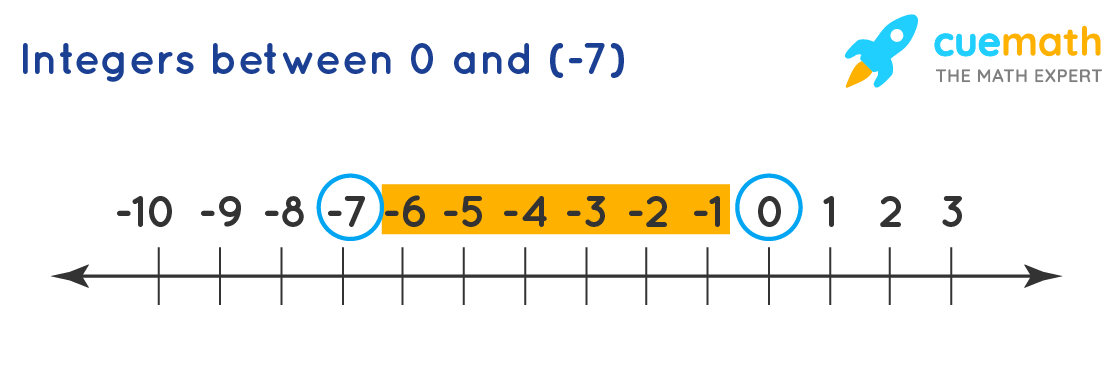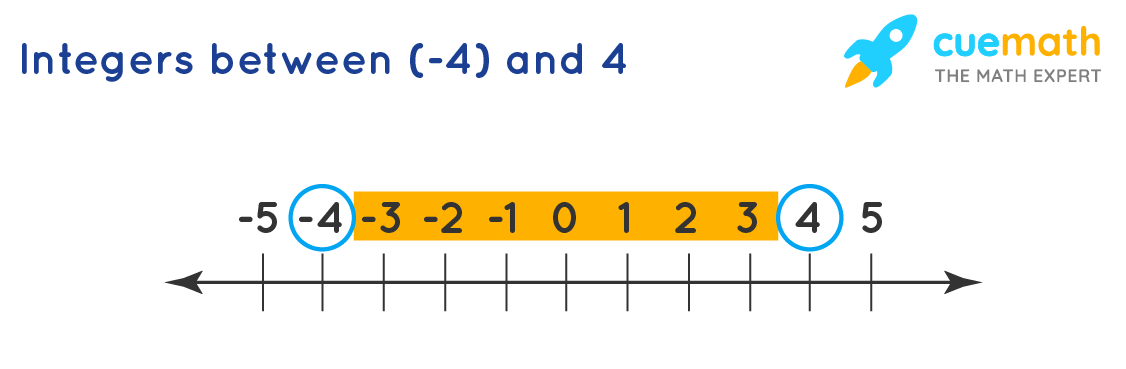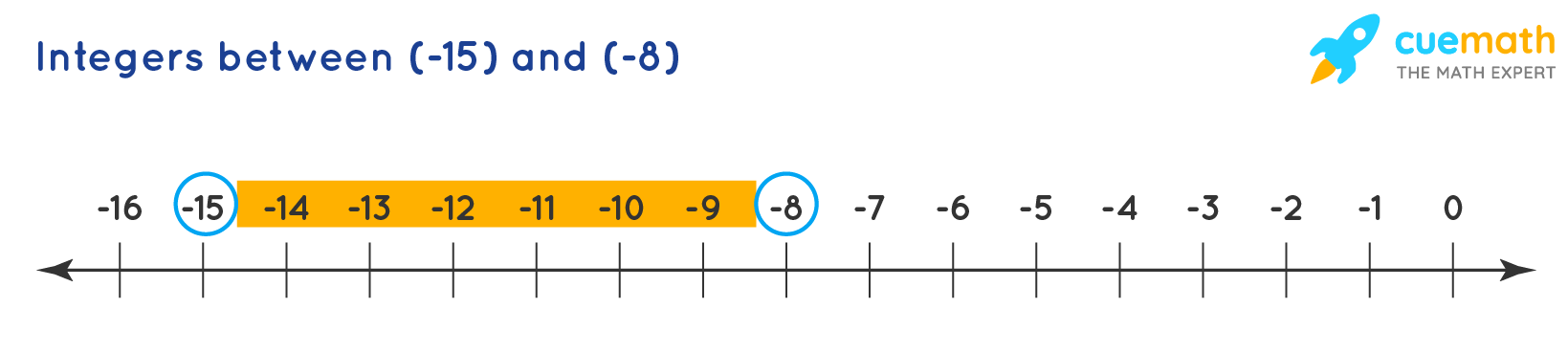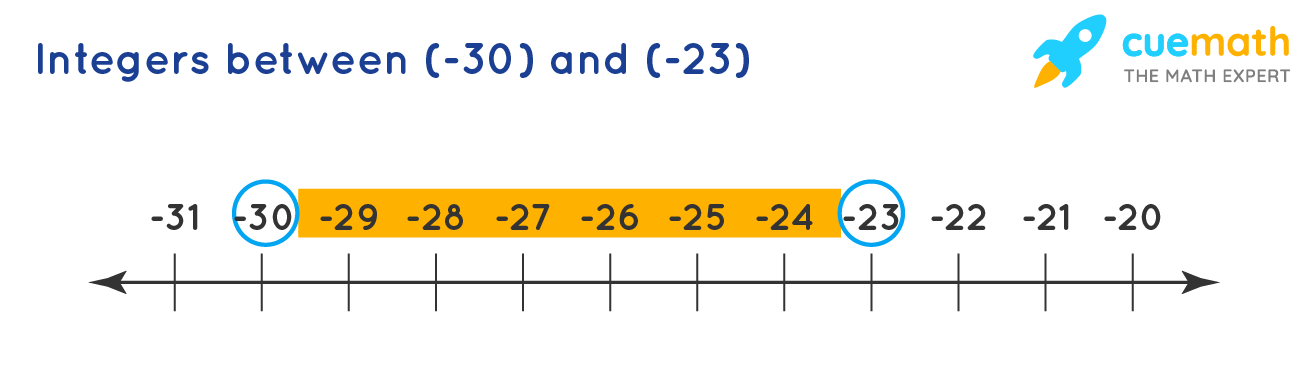# Write All the Integers Between the Given Pairs (Write Them in the Increasing Order):

## (a) 0 and -7   (b) -4 and 4   (c) -8 and -15   (d) -30 and -23

A number line is a visual representation of numbers on a straight line.

## (d) -29, -28, -27, -26, -25, -24

If we observe the given number line, we find that the origin is 0 (zero), which stands in the middle. To the right, there are positive numbers and to the left, there are negative numbers.

## Explanation:

Draw the number line to represent these pairs.

(a)It is clearly from the number line that the integers -6, -5, -4, -3, -2 and -1 between -7 and 0

(b)It is clearly from the number line that the integers -3, -2, -1, 0, 1, 2, and 3 between -4 and 4

(c)It is clearly from the number line that the integers -14, -13, -12, -11, -10, and -9 between -15 and -8

(d)It is clearly from the number line that the integers -29, -28, -27, -26, -25, -24 between -30 and -23